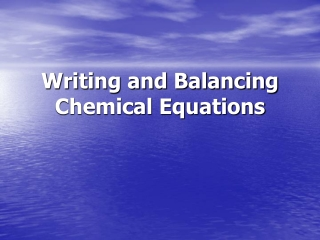DownloadDownload PresentationWriting and Balancing Chemical Equations

# Writing and Balancing Chemical Equations

Download Presentation## Writing and Balancing Chemical Equations

- - - - - - - - - - - - - - - - - - - - - - - - - - - E N D - - - - - - - - - - - - - - - - - - - - - - - - - - -
##### Presentation Transcript

1. Writing and Balancing Chemical Equations

2. Chemical Equations • Chemical reactions result in chemical changes. • Chemical changes occur when new substances are created. • The original substance(s), called reactants, change into new substance(s) called products.

3. Chemical Equations • Chemical reactions can be written in different ways. • A word equation: • Nitrogen monoxide + oxygen  nitrogen dioxide • A symbolic equation: • 2NO(g) + O2(g) 2NO2(g) State of matter • Letters indicate the state of each compound. (aq) = aqueous/dissolved in water (s) = solid ( ) = liquid (g) = gas Coefficients • Indicate the ratio of compounds in the reaction. • Here, there is twice as much NO and NO2 than as is O2. See pages 202 - 203 (c) McGraw Hill Ryerson 2007

4. Conservation of Mass in Chemical Change • Chemical change means new compounds are created. • No new matter is created or destroyed; atoms are just rearranged. • All of the matter in the reactants = all of the matter in the products. • John Dalton, 200 years ago, realized that atoms simply rearrange themselves during chemical reactions. • Number of each atom in reactants = number of each atom in products.

5. Conservation of Mass in Chemical Change • The Law of Conservation of Mass: • In chemical reactions, atoms are neither created nor destroyed. • This law was developed by Antoine and Marie-Anne Lavoisier in the 1700s. • Mass of reactants = mass of products If you could collect and measure all of the exhaust from this car, you would find that mass of reactants (gas + O2) = mass of products (exhaust).

6. Writing and Balancing Chemical Equations • The simplest form of chemical equation is a word equation. • Potassium metal + oxygen gas  potassium oxide • A skeleton equation shows the formulas of the elements/compounds. • A skeleton equation shows atoms, but NOT quantities of atoms. • K + O2 K2O  Is this equation balanced?? See page 206 (c) McGraw Hill Ryerson 2007

7. Writing and Balancing Chemical Equations • A balanced chemical equation shows all atoms and their quantities • Balancing ensures that the number of each atom is the same on both sides of the reaction arrow. • Always use the smallest whole-number ratio. Balance K + O2 K2O • 4K + O2= 2K2O See page 206 (c) McGraw Hill Ryerson 2007

8. Word Equations  Skeleton Equations

9. Counting atoms: 2 methods, same meaning These two diagrams/equations look different, but both represent the same thing

10. How to count atoms

11. Counting Atoms to Balance an Equation • Using the law of conservation of mass, we can count atoms to balance the number of atoms in chemical equations. • Word equation: methane + oxygen  water + carbon dioxide • Skeleton equation: CH4 + O2  H2O + CO2 • To balance the compounds, look at how many atoms of each element occur on each side of the reaction arrow. • Skeleton equation: CH4 + O2  H2O + CO2 1 carbon, 4 hydrogen, 2 oxygen 1 carbon, 2 hydrogen, 3 oxygen • To balance, find coefficients that equate atoms on both sides • Balanced equation: CH4 + 2O2  2H2O + CO2 1 carbon, 4 hydrogen, (2×2) oxygen 1 carbon, (2×2) hydrogen, (2×1)+2 oxygen The same number of atoms must be on each side.

12. Practice: Balance the following chemical equation: Balancing Step 1: * A skeleton equation is simply a representation of the chemical equation before any balancing is done

13. Now the equation is perfectly balanced Balancing equations: Step 2 Balancing Step 2:

14. Strategies for Balancing Equations • Balance chemical equations by following these steps: • Trial and error will work but can be very inefficient. • Balance compounds first and elements last. • Balance one compound at a time. • Only add coefficients; NEVER change subscripts. • If H and O appear in more than one place, attempt to balance them LAST. • Polyatomic ions (such as SO42–) can often be balanced as a whole group. • Always double-check after you think you are finished.

15. Balance the following: • Fe + Br2 FeBr3 • Sn(NO2)4 + K3PO4 KNO2 + Sn3 (PO4)4 • C2H6 + O2 CO2 + H2O 2Fe + 3Br2 2FeBr3 3Sn(NO2)4 + 4K3PO4 12KNO2 + Sn3 (PO4)4 2C2H6 + 7O2 4CO2 + 6H2O